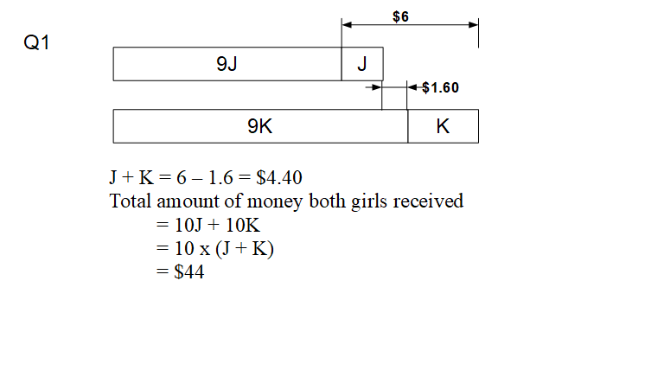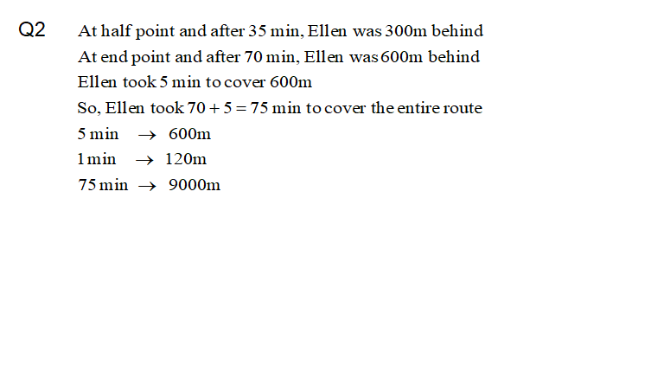# Question

Pls help for the following Qs: thank you.

Kristal and Joy received a sum of money each. If Joy spent 10% of her money, she would have \$6 less than Kristal. If Kristal spent 10% of her in money, she would still have \$1.60 more than Joy. What was the total amount of money that both girls received?

Q2: Dan and Ellen started jogging at the same time along the route. both did not change their speeds throughout. after 35 mins, Dan was at the half way point and Ellen was 300m behind. Dan reached the end point 5 min before Ellen. what was the distance of the route?0 Replies 1 Like0 Replies 1 Like

Kristal and Joy received a sum of money each. If Joy spend 10% of her money, she would have \$6 less than Kristal. If Kristal spend 10% of her money, she would still have \$1.60 more than Joy. What was the total amount of money that both girls received?

If Joy spend 10% of her money,
J ——- 10u – 1u = 9u
K ——- 9u + 6

If Kristal spend 10% of her money,
J ——- 9p – 1.60
K ——- 10p – 1p = 9p

10u ——- 9p – 1.60
10p ——- 9u + 6
1p ——- 0.9u + 0.60
10u ——- 9(0.9u + 0.60) – 1.60 = 8.1u + 3.80
1.9u ——- 3.80
1u ——- 2
10u + 9u + 6 = 19u + 6 = 19 x 2 + 6 = 44

Alternatively,
10u ——- 9p – 1.60
1u ——- 0.9p – 0.16
10p ——- 9u + 6 = 9(0.9p – 0.16) + 6 = 8.1p – 4.56
1p ——- 0.81p – 0.456
0.19p ——- 0.456
1p ——– 0.456/9.19 = 2.40
9p – 1.60 + 10p ——- 19 x 2.40 – 1.60 = 44

Ans : \$44.

Dan and Ellen started jogging at the same time along the route. Both did not change their speeds throughout. After 35 min, Dan was at the halfway point and Ellen was 300m behind. Dan reached the end point 5 min before Ellen. What was the distance of the route?

35 x 2 = 70
70 + 5 = 75
D/E = 75/70 = 15/14
D = 15E/14
35E = 35D – 300
7E = 7(15E/14) – 60
14E = 15E – 120
E = 120
120 x 75 = 9000

Alternatively,
Ellen was 600m and 5 min behind when Dan reached the end point.
Ellen’s speed ——– 600/5 = 120m/min
Time taken for Ellen to complete the route = 35 x 2 + 5 = 75min
Distance of the route ——- 75 x 120 = 9000m

Ans : 9000m.